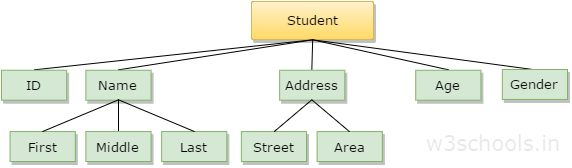# Data Structure Introduction

In the modern world, Data and its information is an essential part, and various implementations are being made to store in different ways. Data are just a collection of facts and figures, or you can say data are values or a set of values that are in a particular format. A data item refers to a single set of values. Data items are then further categorized into sub-items, which are the group of items that are not being called a plain elementary form of items. Let us take an example where the name of the student may be divided into three sub-items, namely: first name, middle name, and last name. But the ID that is assigned to a student would typically be considered as a single item.In the example mentioned above, such as ID, Age, Gender, First, Middle, Last, Street, Area, etc. are elementary data items, whereas (Name, Address) is group data items.

## What is Data Structure?

In computer terms, a data structure is a Specific way to store and organize data in a computer's memory so that these data can be used efficiently later. Data may be arranged in many different ways, such as the logical or mathematical model for a particular organization of data is termed as a data structure. The variety of a specific data model depends on the two factors -

• Firstly, it must be loaded enough in structure to reflect the actual relationships of the data with the real-world object.
• Secondly, the formation should be simple enough so that anyone can efficiently process the data each time it is necessary.

## Categories of Data Structure

The data structure can be subdivided into major types:

• Linear Data Structure
• Non-linear Data Structure

### Linear Data Structure

A data structure is said to be linear if its elements combine to form any specific order. There are two techniques of representing such linear structure within memory.

• The first way is to provide the linear relationships among all the elements represented using linear memory location. These linear structures are termed as arrays.
• The second technique is to provide a linear relationship among all the elements represented by using the concept of pointers or links. These linear structures are termed as linked lists.

The common examples of the linear data structure are:

• Arrays
• Queues
• Stacks

### Nonlinear Data Structure

This structure is mostly used for representing data that contains a hierarchical relationship among various elements.

Examples of Non-Linear Data Structures are listed below:

• Graphs
• the family of trees and